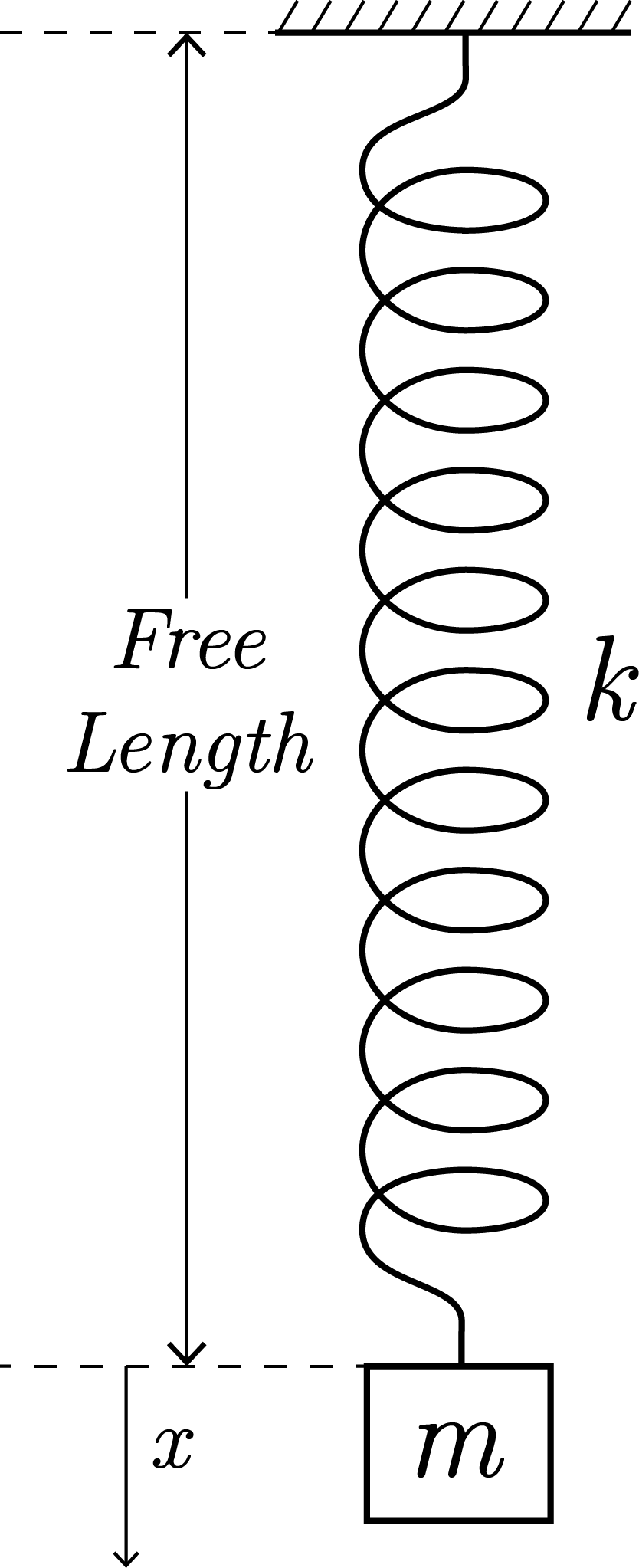Spring and SHM - 1A block of mass $m$ is gently attached to the spring and released at time $t=0$, when the spring has its free length. During subsequent motion of the block, the displacement of the block $x$ with respect to time $t$ is considered. Find $\displaystyle\int_0^3 x ~\mathrm{d}t$

Details and Assumptions

• Neglect air resistance.
• $k$ is the spring constant.
• $m = 10\text{ kg},k = 34 \text{ Nm}^{-1}, g = 9.8 \text{ ms}^{-2}$

×